×

×

AU / Chemistry / CHEM 1030 / What are the states of matter?

# What are the states of matter? Description

CHEM 1030 FINAL EXAM STUDY GUIDE

## What are the states of matter?CHAPTERS 1-3

States of Matter

• Solid: particles tightly packed with no space in between (ice)

• Liquid: particles are packed loosely with some space in between (water at room  temperature)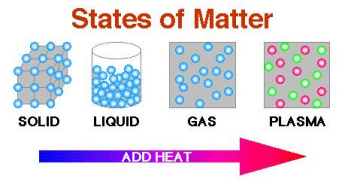• Gas: particles are not packed and roam freely (water vapor)

Mixtures

• Homogenous mixtures: these mixtures are thoroughly mixed to where you cannot tell the  original substances (also called a solution).

## How many elements are there in the periodic table?• Heterogeneous mixtures: these are not thoroughly mixed, and you can often tell what the  original substances were.

Properties

• Quantitative properties: are measured or expressed using a number, ALWAYS ADD  UNITS!

• Qualitative properties: are based mainly on observation  We also discuss several other topics like What is the scope of economics?

• Physical properties: are those, which can be observed/measured without changing the  identity of the substance. Some types of physical properties are: color, melting point,  boiling point, smell, etc.

- Physical changes: are those, which disrupt the state of matter of a substance (the  substance PHYSICALLY changes). Don't forget about the age old question of How the tax burden determined?

• Chemical properties: is a reaction that a substance has when interacting with another  substance.

## Radiation is the transmission of what?- Chemical changes: reactions that result in the total change of the composition of the  substance, so that the original one does not exist anymore.

• Extensive properties: depend on how much matter there is in an object, the more matter  an object has the more its mass is

• Intensive properties: don’t depend on how much matter there is in an object, temperature  and density are 2 types Don't forget about the age old question of What is the concept of perception?

• Mass: the measure of the amount of matter in an object

CHEM 1030 FINAL EXAM STUDY GUIDE

• Weight: the force. which is exerted by an object due to gravity, the weight of an object  varies from place to place since gravity is involved. We also discuss several other topics like Why does the distribution of means have a smaller variance than the population of individuals?
Don't forget about the age old question of What is one of the most important measures of public opinion?

Math

• Significant figures: are the MEANINGFUL digits in a number

• Accuracy: shows how close or exact a measurement is to the real value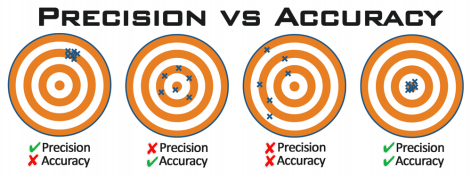• Precision: shows how close a set of measurements are to one another

• Dimensional analysis: also known as the factor label method, is a way of converting units  in problem solving

• Conversion factors: fractions where 2 quantities that mean the same thing (equal to each  other) are put one in the numerator and other in the denominator.

Prefix

Symbol

Meaning

Tera-

T

1×10!"

Giga-

G

1×10!

Mega-

M

1×10!

Kilo-

k

1×10!

Deci-

d

1×10!!

Centi-

c

1×10!!

Milli-

m

1×10!!

Micro-

µ

1×10!!

Nano-

n

1×10!!

Pico-

p

1×10!!"

Don't forget about the age old question of Does rearrangement occur in oxymercuration demercuration?

The Periodic Table

• The periodic table: consists of 118 elements that are grouped according to their physical  and chemical properties.

• Metals: good conductors of heat and electricity

• Nonmetals: bad conductors of heat and electricity

CHEM 1030 FINAL EXAM STUDY GUIDE

• Metalloid: in between metal and nonmetal properties (they are okay conductors of heat  and electricity).

• Group 1A: the alkali metals (Li, Na, K, Rb, Cs, and Fr)

• Group 2A: the alkaline earth metals (Be, Mg, Ca, Sr, Ba, and Ra)

• Group 6A: the chalcogens (O, S, Se, Te, and Po)

• Group 7A: the halogens (F, Cl, Br, I, and At)

• Group 8A: the noble gases (He, Ne, Ar, Kr, Xe, and Rn)

• Groups 1B, 3B-8B: transition elements/transition metals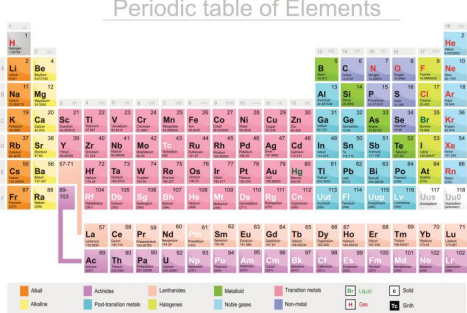• Radiation: is the transmission of energy in the form of waves

• Cathode ray tube: also known as the electron beam, is a glass tube with metal plates on  each end, which has all air, sucked out from it. This device detects electrons using  radiation.

• Cathode: is a negatively charged plate within the cathode ray which produces radioactive  waves called cathode rays.

• Anode: is a positively charged plate within the cathode ray.

• Columb’s law: like charges push against one another and opposite charges come towards  one another.

CHEM 1030 FINAL EXAM STUDY GUIDE

• X-rays: rays that are able to penetrate matter and cause materials to give off a fluoresce  light. They do no have charged particles due to them not being deflected by anything. • Radioactivity: a spontaneous emission of rays that are highly energetic and cannot be  deflected due to anything

Atoms

• Atoms: are the building blocks and smallest units of matter

• Element: contain a unique form of the same atom (each element has a different form of  atoms), which cannot be divided into 2 or more simpler substances

• The atomic number: the number that defines the element, it also represents the number of  protons that are in the nucleus of that atom.

• The atomic mass number: the total number of protons and neutrons that are in the nucleus  of an element’s atom.

• Isotopes: are atoms, which have the same atomic number, but different atomic mass, (the  number of neutrons is different from the number of protons).

• Atomic mass: the mass of an atom in atomic mass units (amu).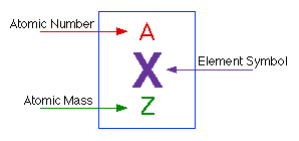The Mole

• Mole: unit of measurement that shows the quantity in any substance that has the same  number of particles (atoms) found in 12.000 grams of carbon 12. It is like a dozen (12) or  a gross (144).

• Avogadro’s number: the number of atoms in 12.000 grams of carbon 12 (6.022 x 10!!) • Molar mass: the mass in grams of one mole of a substance.

Energies

• Kinetic energy: results from motion

• Potential energy: is the energy an object has at a still position

• Chemical energy: energy which is stored in the structural units of chemical substances • Electrostatic energy: energy which results from the interaction of charged particles • Law of conservation of energy: the total amount of energy in the universe is constant, and  it cannot be created or destroyed.

CHEM 1030 FINAL EXAM STUDY GUIDE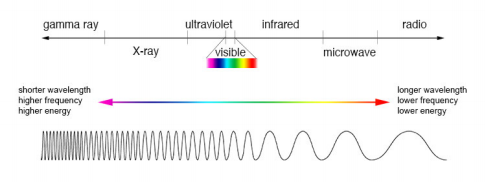• Wavelength (λ): the distance between two peaks of a wave

• Frequency (ν): the number of waves that pass through a certain point in 1 second. • Amplitude: the vertical distance from the middle of the wave to the top of the peak  • Electromagnetic wave: a wave which possess an electric field and magnetic field  component

• Blackbody radiation: the radiation a solid gives off when it is heated

• Photoelectric effect: a theory which states that when electrons are exposed to light on a  metal surface, they are ejected from that surface

• Threshold frequency: the minimum of light that can cause electron emission  • Photons: little particles of light

• Emission spectra: the heat given off a piece of sample material when it is energized using  either thermal or other form of energy

• Line spectrum: the emission of light only at certain wavelengths

• Ground state: as an electron gets closer to the nucleus it becomes more negative, and at  its most stable energy point

• Excited state: the energy state, which is more positive. The electron jumps from the  ground state to the excited state.

Principle Quantum Numbers

• The principle quantum number (n): the main energy level/shell, the higher the “n” is, the  further away the level/shell is away from the nucleus.

• The angular quantum number (ℓ): this describes the shape of the orbital. 0 and n-1 - If n=2 then ℓ=0 and 1

• The magnetic quantum number (mℓ): this describes the orientation.

- If n=3 then mℓ= -3, -2, -1, 0, 1, 2, 3

• The spin quantum number (m!): this describes the spin, -1/2 or +1/2.

• Orbitals Types

- ℓ=0 is an “s” orbital

CHEM 1030 FINAL EXAM STUDY GUIDE

- ℓ=1 is a “p” orbital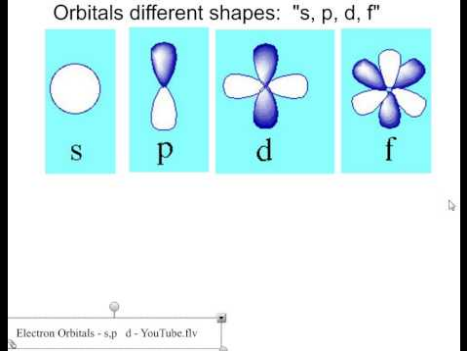- ℓ=2 is a “d” orbital

- Electron capacity for each shell is 2n!

o n=1???? 2

o n=2???? 8

o n=3???? 18

o n=4???? 32

CHAPTERS 4-6

Periodic Trends

• Atomic radius: the distance between 2 nuclei divided by 2.

- Trends on the periodic table for atomic radius

o As you go right or up, the atomic radius gets smaller.

o As you go left or down, the atomic radius gets bigger.

• Shielding: the nucleus attracts the electrons in the outer most shell towards it; however,  the electrons in the shells that are in between the nucleus and the outer most shell, push  them back.

• Ionic radius: the radius of an atom’s cation (+) or an anion (-).

- The ionic radius of a cation would be smaller than the ionic radius of an anion, due to  an anion having more electrons.

- Example: a regular carbon atom has the electron configuration of 1s!2s!2p! and 4  electrons in its valence shell.

o A carbon cation (+1 charge) will have the electron configuration of 1s!2s!2p! and 3 electrons in its valence shell due to having 1 less electron to make it a  cation.

o A carbon anion (-1 charge) will have the electron configuration of 1s!2s!2p! and  5 electrons in its valence shell due to having 1 extra electron to make it an anion. • Ionization energy: the energy it takes to remove an electron (can also be called  cationization—since you’re making the atom more positive by removing the electrons). - Trends on the periodic table for ionization energy

o As you go right or up, the energy required to remove an electron becomes very  hard—more energy required.

o As you go left or down, the energy required to remove an electron becomes much  easier—less energy required.

• Effective nuclear charge: the nuclear energy felt by an electron due to the effect of  shielding from the inner shell electrons. Z!"" = proton # (Z) – inner shell electrons (S)

CHEM 1030 FINAL EXAM STUDY GUIDE

- Example: find the effective nuclear charge for magnesium

o Protons: 12

o Inner shell electrons: (subtract valence electrons from proton number) 10 o Z!"" = 12-10, so the effective nuclear charge is 2

- Trends on the periodic table for effective nuclear charge.

o As you go right or up, the effective nuclear charge increases.

o As you go left or down, the effective nuclear charge decreases.

• Electron affinity: the energy that is released from an atom that is in the gas phase, when it  accepts an electron.

- Trends on the periodic table for electron affinity

o As you go right or up, the electron affinity increases.

o As you go left or down, the electron affinity decreases.

• Electronegativity: the ability for an atom to draw shared electrons to itself. - A bond where the electronegativity between the atoms is less than 0.5 is said to be  nonpolar.

- A bond where the electronegativity between the atoms is between 0.5 and 2.0, is said to  be polar covalent.

- A bond where the electronegativity between the atoms is more than 2.0 is said to be ionic.  - Trends on the periodic table for electronegativity

o As you go right or up, the electronegativity increases.

o As you go left or down, the electronegativity decreases.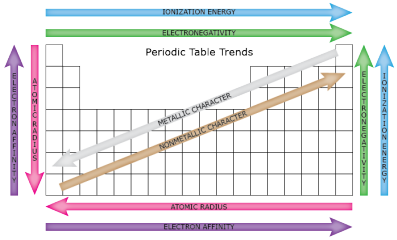• Molecular mass: the sum of the atomic masses of the atoms in the elements, which make up a  specific molecule.

- Example: H!O???? 2(1.008 amu)+16.00 amu=18.02 amu

CHEM 1030 FINAL EXAM STUDY GUIDE

• The octet rule: atoms will gain or lose electrons to get 8 electrons on their valence shell. They  want to achieve the same stability as the noble gasses.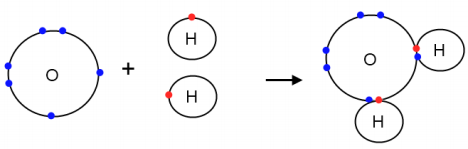Naming

• Ionic compound: consists of anions and cations that are held together by ionic bonding. - Naming ionic compounds

o If the charges are the same, all you have to do is write the first element as is, and then  replace the ending with –ide off the second element

▪ Example: Na! and F! will be “sodium fluoride”

o If charges are different, then you first have to balance out the charges, and then  proceed with using the same rules as if the charges were the same.

▪ Example: Al!!and O!! you will first write the compound as Al!O! so that the  charges are balanced, then you will write the first element as is, and add –ide to  the ending of the second element, so it will be aluminum oxide

o If the compound contains a transition metal, then you will chose the charge of the  transition metal to match the charge of the second element. Then, you will write the  first element as is, then write the charge of the transition element in roman numerals,  and add –ide to the second element.

▪ Example: SnI! you will first notice that the subscript for iodine is 2, so that will  tell you the charge needed for tin, which is also 2. You will write the compound  as tin (II) iodide

• Binary compound: consists of a metal and a nonmetal.

- Naming binary compounds

o You use the same rules as ionic compound naming.

• Covalent compound: consists of 2 nonmetals

- Naming covalent compounds

o When naming covalent compounds you use the subscripts of each element as a prefix,  so if the subscript on an element is 2 you would add “di” before the element name,  the second element also gets an –ide to replace the ending.

▪ Example: N!O would be written as dinitrogen monoxide

• Polyatomic ions: a compound that has 2 covalently bonded atoms.

- Example: BrO!!???? bromate, PO!!!???? phosphate

• Oxoanions: polyatomic ions that have more than one oxygen atom.

- Naming Oxoanions

CHEM 1030 FINAL EXAM STUDY GUIDE

o Ions with one more oxygen atom than the –ate ion, has per at the beginning and -ate  at the end.

▪ Example: CIO!!???? perchlorate ion

o Ions with one less oxygen atom than the –ate anion, has –ite at the end.

▪ Example: CIO!!???? chlorite ion

o Ions with 2 less oxygen atoms than the –ate ion, has hypo at the beginning and –ite at  the end.

▪ Example: CIO!???? Hypochlorite ion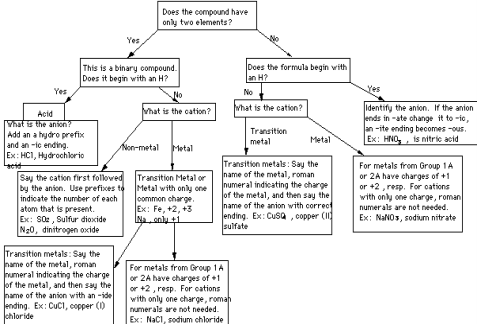CHAPTERS 7-9

VSPER Model

• VSPER model: the way molecular geometry is predicted.

- Electron domain geometry: the arrangement of electrons around the central atom. - Molecular geometry: the arrangement of atoms that are bonded.

- Bond angle: the angle between 2 electron bonds that are next to each other.  - Electrons repel each other and are found in domains—lone pairs, single bonds, double  bonds, and triple bonds.

o 2 electron domains: linear shape

o 3 electron domains: trigonal planar shape

CHEM 1030 FINAL EXAM STUDY GUIDE

o 4 electron domains: tetrahedral shape

o 5 electron domains: trigonal bipyramidal shape

o 6 electron domains: octahedral shape

- When the central atom in a molecule has 1+ lone pairs, then the electron domain  geometry and molecular geometry are not the same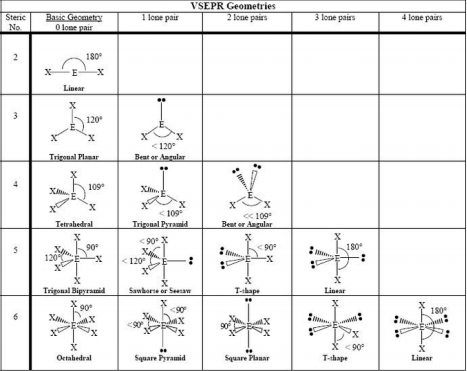Acids and Bases

• Acids and Bases

- Arrhenius acid: ionizes in water to produce H+ ions.

- Arrhenius base: dissociate sin water to produce OH- ions.

- Bronsted acid: proton (H+) donor.

o Hydronium ion: (H3O+) formed by protons donated by bronsted acids. - Bronsted base: proton (H+) acceptor.

- Monoprotic acid: has a proton to donate (only 1).

- Polyprotic acid: has 1+ acidic H atom.

- Diprotic acid: has 2 acidic H atoms.

• Neutralization reaction: the reaction between acids and bases.

CHEM 1030 FINAL EXAM STUDY GUIDE

Redox Reactions

• Redox reaction: a chemical reaction in which electrons are transferred from one reactant, to  the other reactant.

- Oxidation: loss of electrons.

- Reduction: gain of electrons

- Here is a way to remember: OIL RIG (Oxidation Is Loss, Reduction Is Gain) • Oxidation number: the charge an atom would have if the electrons were all transferred.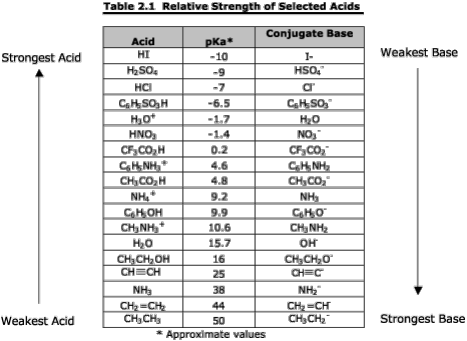Chemical Equation

• Balancing equations: adding coefficients to different parts of a chemical equation, so that you  have the same number of each element on both sides.

- ALWAYS make sure an equation is balanced before doing any kind of chemistry  problem!!!

• There are 3 reaction types.

- Combination reaction: when 2+ reactants combine to form 1 product.

- Decomposition: when 2+ products form from 1 reactant.

- Combustion: when a substance burns with oxygen present, and produces carbon dioxide  and liquid water

Intermolecular Forces

• Intermolecular forces: the forces between molecules that are next to each other.  • Dipole-dipole interactions: the forces between polar molecules.

CHEM 1030 FINAL EXAM STUDY GUIDE

• Hydrogen bonding: a type of dipole-dipole interaction that occurs only in molecules with  hydrogen bonded to a very electronegative atom.

• Dispersion forces: are due to coulombic attractions between instantaneous dipoles of non polar molecules.

• Ion dipole interactions: coulombic attractions that happen between +/- ions and polar  molecules.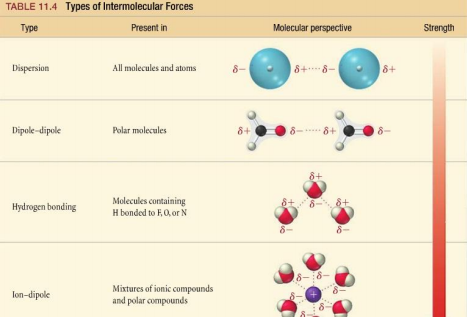Limiting/Excess Reactants

• Limiting reactants: the reactant that is used up first in a chemical reaction.

• Excess reactants: the reactants that are left over (the extras).

• Theoretical yield: how much product forms from all the limiting reactants.

• Actual yield: how much product you actually get from the reaction.

• Percent yield: (actual yield/theoretical yield) x 100%

Electrolytes

• Electrolytes: substance, which dissolve in water to form a solution that can conduct  electricity.

- Dissociation: when an electron breaks up into constituent ions.

o Strong electrolyte: and electrolyte that completely dissociates.

o Weak electrolyte: a compound that produces ions when dissolving, but is in a solution  in which it exists as molecules that aren’t ionized.

• Nonelectrolyte: (opposite of electrolyte) a substance that dissolves in water to form a solution that can’t conduct electricity.

CHEM 1030 FINAL EXAM STUDY GUIDE

Valence Bond Theory

• Valence bond theory: states that when atomic orbitals overlap, they share electrons. • Hybridization: occurs when atomic orbitals mix to form new ones. The new ones have the  same capacity of electrons as the old ones, but the properties and energies are different.  Hybridization is essentially the average. There are 2 kinds of bonds that form. - Sigma bonds

- Pi bonds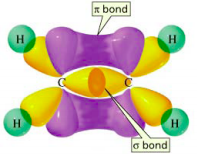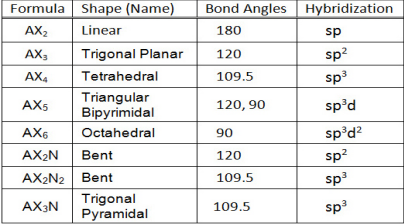Ionic Equations

• Ionic equation: compounds that exist as ions in a solution are then represented as those ions.  • Net ionic equation: an equation that has only the species that are involved in the reaction. Solutions/Reactions

• Solution: a mixture of 2+ substances.

• Solvent: the largest amount of substance in a solution. This dissolves the solute. • Solute: all the other substances that are present. This dissolves in the solvent. • Precipitation reaction: a chemical reaction where precipitate forms.

CHEM 1030 FINAL EXAM STUDY GUIDE

• Hydration: when water molecules steal ions from ionic solids around them so that the solids  dissolve.

• Solubility: the most solute you need to dissolve a certain amount of solvent at a certain  temperature.

CHAPTERS 10-11

Thermochemistry

• Thermochemistry: the study of heat and transfer of thermal energy in chemical reactions.  - Heat and temperature aren’t the same thing.

o Heat is the transfer of energy, and can be absorbed or released.

o Joules (J) is the unit

- 1 calorie= 4.184 joules

• Exothermic process: occurs when heat is transferred from the system to the surroundings. • Endothermic process: occurs when heat is transferred from the surroundings and into the  system.

Thermo Dynamics

• Thermodynamics: the study of the interconversion of heat and other kinds of energy. • Open system: can exchange mass and energy with the surroundings.

• Isolated system: cannot exchange energy or mass outside of the system’s boundaries, this can  also be referred to as a closed system.

• State functions:

• The 1st Law of Thermodynamics: energy can be converted from one form to another, but  cannot be created or destroyed.

Enthalpy

• Enthalpy: the thermodynamic function of a system.

- Enthalpy and heat are interchangeable.

• Enthalpy of a reaction: the difference between the enthalpies of the products and the  enthalpies of the reactants.

• The standard enthalpy of formation: this is defined as heat change that results when 1 mole of  a compound is formed from its constituent elements in their standard states.  • The standard enthalpy of reaction: defined as the enthalpy of a reaction carried out under  standard conditions.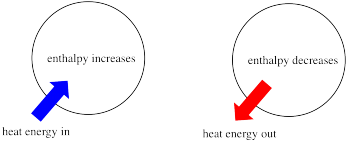CHEM 1030 FINAL EXAM STUDY GUIDE

Calorimetry

• Calorimetry: the measurement of heat changes.

• Specific heat: the amount of heat that is required to raise the temperature of 1 gram of  substance 1 degree Celsius.

• Heat capacity: the amount of heat that is required to raise the temperature of an object by 1  degree Celsius.

• Hess’s Law: states that since enthalpy is a function, the change in enthalpy that occurs when  reactants are converted to products in a reaction is the same whether the reaction takes place  in a series of steps or just one step.

Gasses

• There are 4 gas properties.

- Gas takes the shaped and the volume of the container it is in.

- Gasses are compressible.

- Gas densities are smaller than liquid and solid densities.

- Gasses form solutions with one another.

• Kinetic molecular theory: explains how the molecular nature of gasses gives rise to the  gasses’ macroscopic properties. There are 4 basic assumptions of this theory. - A gas has particles that are very spread apart.

- Gas molecules are always randomly moving.

- Gas molecules do not exert any type of force on one another.

- The average kinetic energy of gas molecules in a sample is proportional to the absolute  temperature.

• Decreasing gas volumes increase the collision frequency.

• Gas pressure increases as collision frequency increases.

• Root mean square speed: the speed of a molecule with the average kinetic energy in a gas  ample.

• Diffusion: when random motion and frequent collisions result in the mixing of gasses. • Effusion: the escaping of gas molecules from a container to a vacuumed region. • Graham’s law: states the diffusion/effusion rate of a gas is inversely proportional to the  square root of its molar mass.

• Ideal gas: hypothetical gas sample, in which the pressure-volume-temperature behavior is  predicted by the ideal gas equation.

CHEM 1030 FINAL EXAM STUDY GUIDE

Pressure

• Pressure: the force that is applied per unit area.

- The SI unit of force is the newton (N).

- The SI unit of pressure is the pascal (Pa).

• Manometer: a device that measures pressures other than atmospheric pressures.  • Standard temperature and pressure: a set of conditions where pressure is 1 atm, temperature  is 0 degrees celcius, and the volume occupied by 1 mole of an ideal gas is 22.41 L. • Dalton’s law for partial pressure: the total pressure exerted by a gas mixture is the sum of the  partial pressures exerted by each component of the mixture.

Page ExpiredIt looks like your free minutes have expired! Lucky for you we have all the content you need, just sign up here